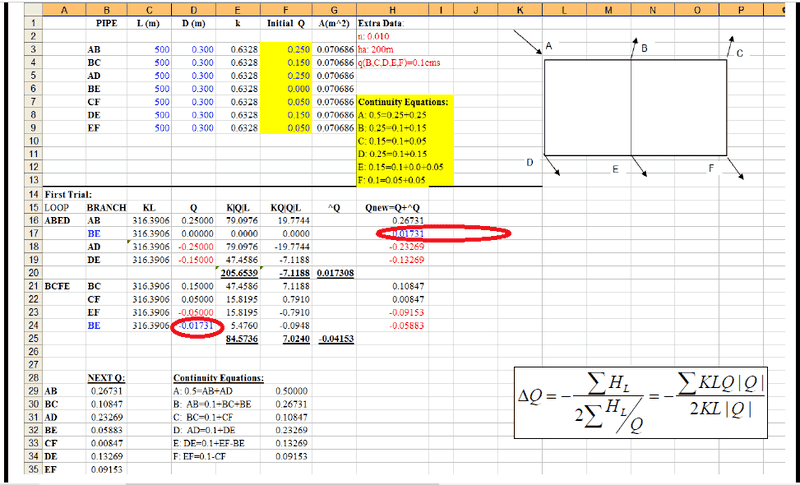# Using corrcted value for Hardy Cross method?

• foo9008

## Homework Statement

i have several example of hardy cross problem involve 2 loops , for the junction that divide 2 loops , is it necessary to use the corrected value for the junction on second loop for the first trial ? or just use the initial assumption will do ?
using this example , the BD is the junction that divide 2 loops , should we use the corrected value 0.8 -(-0.46) =1.26 or stick to the original assumption (0.8) just like the author ?

in the second example here , the author use the corrected value (0.01731) for junction BE in the second loop instead of 0.0## The Attempt at a Solution

i think in the first example , we should use he corrected value 0.8 -(-0.46) =1.26 instead of 0.8

which is correct ?

which is correct ? with or without correction ?